## QUESTIONS

SECTION I (50 marks)

Answer all questions in this section in the spaces provided.

1. Ntutu had cows, sheep and goats in his farm. The number of cows was 32 and the number of sheep was 12 times the number of cows. The number of goats was 1344 more than the number of sheep. If he sold ¾ of the goats. Find the number of the goats that remained.    (4mark)
2. Use the prime factors of 1764 and 2744 to evaluate                      (3 marks)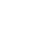3. The mass of a solid cone of radius 14 cm and height 18 cm is 4.62 kg. Find its density in g/cm3. (3 marks)
4. The figure below represents a triangular prism ABCDEF. X is a point on BC.1. Draw a net on the prism (2 marks)
2. Find the distance DX. (1 mark)
5. A business man makes a profit of 20% when he sells a carpet for Ksh 36 000. In a trade fair he sold one such carpet for Ksh 33 600. Calculate the percentage profit made on the sale of the carpet during the trade fair. (3 marks)
6. Simplify   (3 marks)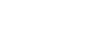7. The area of a sector of a circle, radius 2.1 cm, is 2.31 cm2. The arc of the sector subtends an angle θ, at the centre of the circle. Find the value of in radians correct to 2 decimal places. (2 marks)
8. Expand and simplify  (x + 2y)2 - (2y - 3)2   (2 marks)
9. A plane leaves airstrip L and flies o a bearing of 0400 to airstrip M, 500 km away. The plane then flies on a bearing of 3160 to airstrip N. The bearing of N from L is 3500. By scale drawing, determine the distance between airstrips M and N. (4 marks)
10. The sum of interior angles of a regular polygon is 18000. Find the size of each exterior angle. (3 marks)
11. A cow is 4 years 8 months older than a heifer. The product of their ages is 8 years. Determine the age of the cow and that of the heifer. (4 marks)
12. Solve 4 ≤ 3x - 2 < 9 + x hence list the integral numbers that satisfies the inequality.(3 marks)
13. The figure below shows a rectangular container of dimensions 40 cm by 25 cm by 90 cm. A cylindrical pipe of radius 7.5 cm was fitted in the container as shown.Water is poured into the container in the space outside the pipe such that the water level is 80% the height of the container. Calculate the amount of water, in litres, in the container correct to 3 significant figures. (4 marks)
14. A minor arc of a circle substends an angle of 1050 at the centre of the circle. if the radius of the circle is 8.4 cm, find the length of the major arc. ( Take π = 22/7) (3 marks)
15. Twenty five machines working at the rate of 9 hours per day can complete a job in 16 days. A contractor intends to complete the job in 10 days using the similar machines working at the rate of 12 hours per day. Find the number of machines the contractor requires to complete the job.    (3 marks)
16. Points A (-2, 2) and B (-3, 7) are mapped onto A' (4, -10) and B' (0, 10) by an enlargement. Find the scale factor of the enlargement. (3 marks)

SECTION II (50 marks)
Answer any five questions in this section in the spaces provided.

1. A line L passes through points (-2,3), and (-1, 6) and is perpendicular to line P at ( -1, 6).
1. Find the equation of L. (2 marks)
2. Find the equation of P in the form ax + by - c, where a, b and c are constants.   (2 marks)
3. Given that another line Q is parallel to L and passes through point (1, 2) find the x and y intercepts of Q.  (3 marks)
4. Find the point of intersection of lines P and Q. (3marks)
2. The lengths, in cm, of pencils used by pupils in a standard one class in a certain day were recorded as follows
3 7 9 9 20 14 10 6 8 13
14 3 17 13 8 12 5 15 14 15
7 12 11 6 10 19 9 14 6 9
10 16 13 9 12 11 10 7 10 11

1. Using a class width of 3, and starting with the shortest lengths of the pencils, make a frequency distribution table for the data. (2 marks)
2. Calculate ;
1. The mean length of the pencils. (3 marks)
2. The percentage of pencils thst were longer than 8 cm but shorter than 15 cm. (2 marks)
3. On the grid provided, draw a frequency polygon for the data.  (3 marks)
3. The figure below represents a speed time graph for a cheetah which covered 825 m in 40 seconds.1. State the speed of the cheetah when recording of its motion started. (1 mark)
2. Calculate the maximum speed attained by the cheetah. (3 marks)
3. Calculate the acceleration of the cheetah in:
1. The first 10 seconds; (2marks)
2. The last 20 seconds. (1 mark)
4. Calculate the the average speed of the cheetah in the first 20 seconds. (3 marks)
4. The figure below shows  a right pyramid VABCDE. The base ABCDE is a regular pentagon.
AO = 15 cm and VO = 36 cm.Calculate:
1. The the area of the base correct to 2 decimal places;  (3 marks)
2. The length of AV;  (1 mark)
3. The surface area of the pyramid correct to 2 decimal places; (4 marks)
4. The volume of the pyramid correct to 4 significant figures. (2 marks)
5. Complete the table below for the function y = x2 - 3x + 6 in the range  -2 ≤ x ≤ 8.   (2 marks)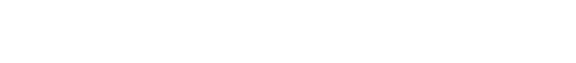1. Use the trapezium rule with 10 strips to estimate the area bounded by the curve, y = x2 - 3x + 6, the lines x = -2, x = 8 and the x -axis. (3 marks)
2. Use the mid-ordinate rule with 5 strips to estimate the area bounded by the curve, y = x2 - 3x + 6, the lines x = -2, x = 8 and the x -axis. (2 marks)
3. By integration, determine the actual area bounded by the curve y = x2 - 3x + 6, the lines x = -2, x = 8 and the x -axis. (3 marks)

1. Using a pair of compasses and a ruler only, construct;
1. Triangle ABC in which AB = 5 cm. <BAC = 300 and angle ABC = 1050; (3 marks)
2. A circle that passes through the vertices of the triangle ABC. Measure the radius. (3 marks)
3. The height of triangle ABC with AB  as the base. Measure the height. (2 marks)
2. Determine the area of the circle that lies outside the triangle correct to 2 decimal places. (2 marks)
6. The figure below represents a piece of land in the shape of the quardilateral in which AB = 240 m, BC = 70 m, CD = 200 m, <BCD = 1500 and < ABC = 900.Calculate;
1. The size of <BAC correct to 2 decimal places; (2 marks)
2. The length of AD correct to one decimal place. (4 marks)
3. The area of the piece of land, in hectares, correct to 2 decimal places. (4 marks)
7. The equation of a curve is given by y = x3-4x2-3x .
1. Find the value of y when x = -1. (1 mark)
2. Determine the stationary points of the curve. (5 marks)
3. Find the equation of the normal to the curve at x  = 1.  (4 marks)## MARKING SCHEME

1. Cows = 32
Sheep = 32 × 12
= 384
Goats = 384 + 1344
= 1728
Number of goats that remained
= 1 × 1728
4
= 432
2.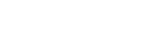=2 × 3 × 7
2 × 7
= 3
3. Volume = 1 × 22 × (14)2 × 18
3    7
= 3696 cm3
Density = 4.62 × 1000
3696
= 1.25 g/cm3
4.DX = 5.3 ± 0.1
√ measurements and angles
√ complete net (labelled)
5. C.P. for carpet
= 36000 × 100
120
= 30000
= 33600 - 30000 × 100
30000
= 12%
6.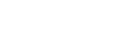= 27 × 25
9
= 75
√ manipulation of all indices or equivalent
√ simplification
7. θ × π × 2.1 × 2.1 = 2.31

θ  = 2.31 × 2
2.1 × 2.1
= 1.05º
8. (x + 2y)2 - (2y - 3)2
= (x2 + 4xy + 4y2) - (4y2 - 12y + 9)
= x2 + 4xy + 12y - 9
9.Distance MN = 6.8 × 100
= 680 km
√ location of M
√ location of N
10. (2n - 4) ×  90 = 1800
180n = 2160
n = 12
size of each exterior angle
= 360 = 30º
12
11. let age of cow be x years
therefore: x(x - 42/3) = 8
3x2 - 14x - 24 = 0
(3x + 4) (x - 6) = 0
x = 6 or - 4
3
Age of cow = 6 years
Age of heifer = 11/3 years
12. 4 ≤ 3x - 2 < 9 + x
4 ≤ 3x - 2      3x - 2 < 9 + x
6 ≤ 3x            2x < 11
x ≥ 2           x < 51/2
therefore: 2 ≤ x < 51/2
Integral values
2, 3, 4, 5
13. Volume of water in container
= 80 × 90(40 × 25 -  π × 7.52)
100
= 59276.54975
59276.54975
1000
= 59.3
14. Angle for major arc = 360 - 105
= 255º
Length of arc = 255 × 2 × 8.4 × 22
360                    7
= 37.4 cm
15. Amount of work = 25 × 16 × 9
Machines required
= 25 × 16 × 9
12 × 10
= 30 M1
16.= 4
17.
1. Equation of L
-1 - 2
= 3
equation = y - 6 = 3
x + 1
= y - 3x = 9
2. equation of P
= y - 6 =- 1
x + 1     3
3y + x = 17
3. equation of Q
= y - 2 = 3
x - 1
y = 3x - 1
x intercept
when y = 0 = x = 1
3
y intercept
when x = 0 =  y =-1
4. Intersection of lines P and Q
3y + x = 17..(i)
y - 3x =- 1.. (ii)
3y + x = 17
3y - 9x =- 3
10x = 20 = x = 2
subset 3y + 2 = 17 = y = 5
therefore: point of intersection (2, 5)
18.
1.
 class 3-5 6-8 9-11 12-14 15-17 18-20 frequency 3 8 13 10 4 2
2.
1. mean length = Σfx
Σf
= 4 × 3 + 7 × 8 + 10 × 13 + 13 × 10 + 16 × 4 + 19 × 2
40
= 10.75
2. = 23 × 100
40
= 57.5%
3.19.
1. 15 m/s
2. maximum speed
1 × (15 + h) × 10 + 1 (10 + 30)h = 825
2                             2
75 + 5h + 20h = 825
25h = 750
h = 30 m/s
3.
1. = 30 - 15
10
= 1.5 m/s2
2. = 0 - 30 =-1.5 m/s2
20
4. [1 (15 + 30) × 10 + 10 × 30] ÷ 20
2
= (225 + 300) ÷ 20
= 26.25 m/s
20.
1. base area
= 1 × 15 × 15 sin 72 × 5
2
= 534.97
2. Length AV
=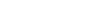3. Area of triangular faces:
AB     =   15
sin 72      sin 54
AB = 15 sin 72
sin 54
= 17.63
therefore: area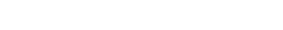= 334.89
Total area = 334.89 × 5 + 534.97
= 2209.42
4. volume of pyramid
= × 534.97 × 36
3
= 6419.63 cm2
= 6420 (4 s.f.)
21.
1.
 x -2 -1 0 1 2 3 4 5 6 7 8 y 16 10 6 4 4 6 10 16 24 34 46
2. Area using trapezium rule
= 1/2 × 1[16 + 46 + 2(10 + 6 + 4 + 4 + 6 + 10 + 16 + 24 + 34)]
= 1/2 [62 + 2(114)]
= 145
3. Area using mid-ordinate rule
= 2 × (10 + 4 + 6 + 16 + 34)
= 140
4. Area using integration method22.
1.
1.2. radius = 3.5 ± 0.1
3. height construction
height = 3.4 ± 0.1
2. area of circle outside triangle
= π × 3.52 - 1/2 × 3.4 × 5
= 29.98
23.
1.  tan θ = 70
240
= 0.2917
θ = 16.26º
2. AC = √702 + 2402
= 250 m
angle ACD = 150º - (90º - 16.26º)
= 76.26º
AD2 = 2002 + 2502 - 2 × 200 × 250 cos 76.26
AD = √40000 + 62500 - 100000 cos 76.26º
= 280.6
3. Area of plot
= 1/2 × 240 × 70 + 1/2 × 250 × 200 sin 76.26º
= 8400 + 24284.59
= 32684.59 m2
= 32684.59
10000
= 3.27 ha
24.
1. Value of y when x =-1
y =-1 - 4 + 3 =-2
2. Stationary points
dy = 3x2 - 8x - 3
dx
for stationary points
3x2 - 8x - 3 = 0
(3x + 1)(x - 3) = 0
x =- 1 or x = 3
3
when x =- 1 , y = 14
3         27
when x = 3 , y =-18
therefore:  stationary points
- 1 ,  14
3    27
and (3, -18)
3. Equation of normal to curve:
gradient of tangent at x = 1
dy = 3 - 8 - 3 =- 8
dx
= 1
8
therefore: equation of normal at x = 1
y + 6 = 1
x - 1     8
y + 6 = 1 x - 1
8      8
y = 1 x - 6 1
8         8

#### Download KCSE 2014 Mathematics Alternative A Paper 1 Questions with Marking Scheme.

• ✔ To read offline at any time.
• ✔ To Print at your convenience
• ✔ Share Easily with Friends / Students